# 故障服务定位算法综述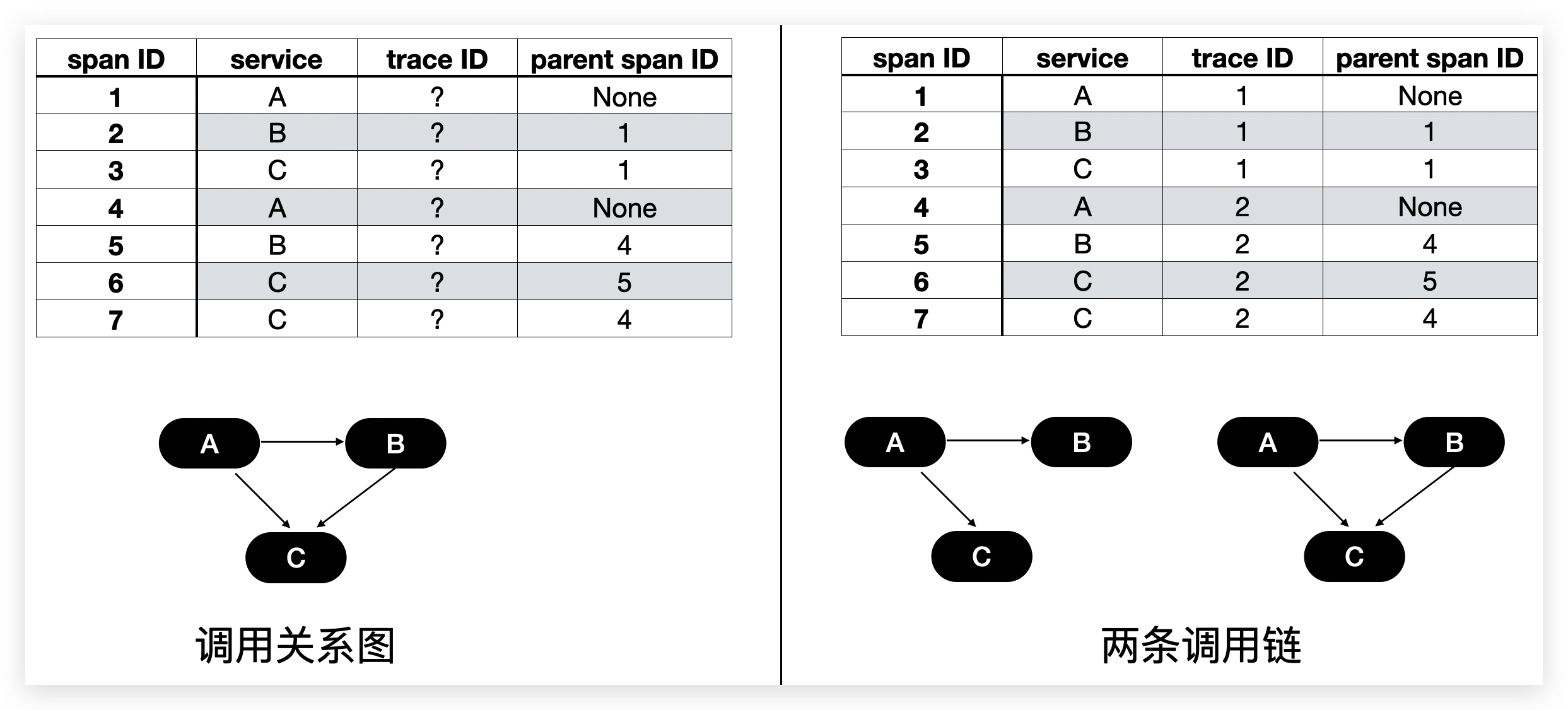1. 只有每个节点的指标信息，或者节点之间的调用信息。[1, 2, 3, 4, 5, 6, 9, 11, 14, 15]
2. 有全局调用链ID可以串起来不同的单次调用。 [7, 8, 12]

### 基于随机游走的方法¶

#### MonitorRank¶

##### 背景¶

MonitoRank把系统的服务分成三类：

1. 前端服务，负责接收用户的请求以及进一步调用下游请求以完成用户的请求。
2. 应用服务，负责真正处理用户请求的逻辑
3. 数据服务，负责提供经过包装的数据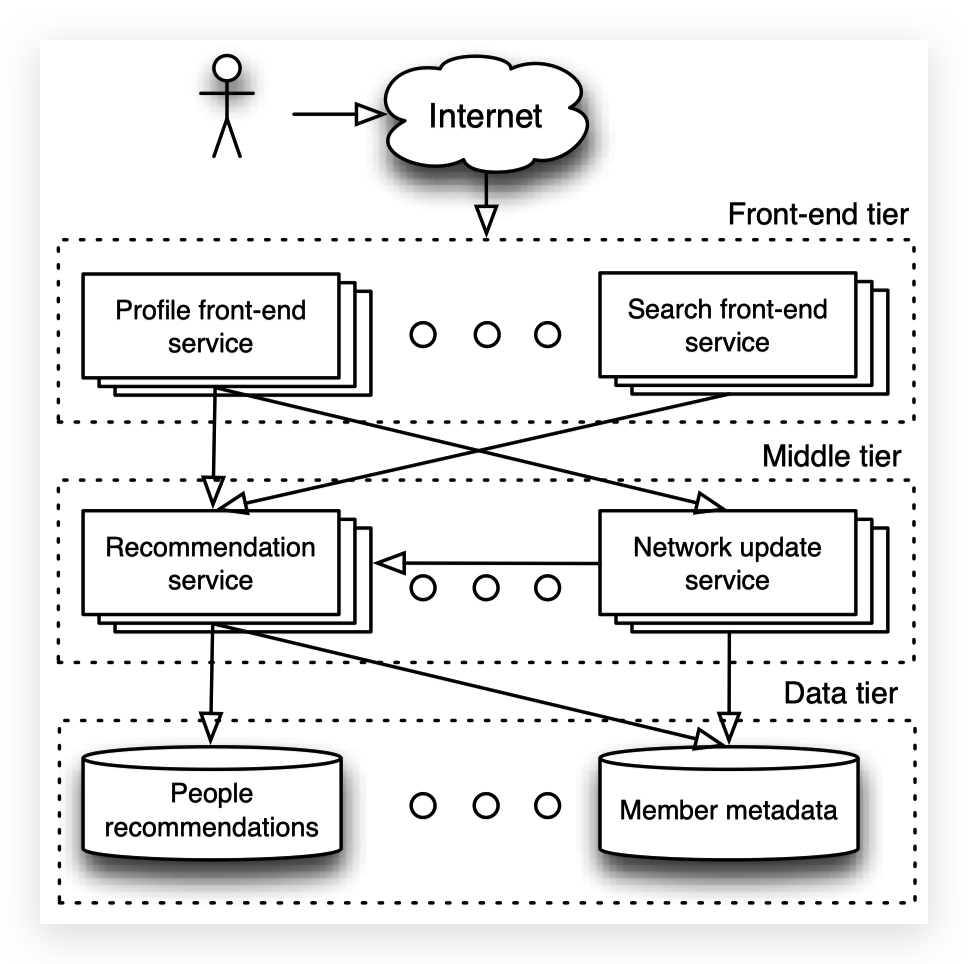MonitorRank和我们研究的其他很多文献一样，只聚焦在异常发生之后的故障定位，和大量的异常检测的相关工作区分开来了。

##### 故障定位¶

MonitorRank基本思想是，一个节点的指标$$m$$和异常前端节点$$v_{fe}$$的指标$$m$$的相关性表示了该节点是根因的可能性。

MonitorRank假设在许多次随机游走过程中，被访问越多的节点越可能是根因，即被访问的概率就是根因排序依据的分数。

$P_{ij}=\frac{A'_{ij}}{\sum_{k}A'_{ik}},\\ A'_{ij}=\begin{cases} A_{ij}S_{j}+A_{ji}S_{i} & i\neq j \\ \max(0, S_i - \max_{k:e_{jk}\in E}S_k) & i = j \neq fe \end{cases}$

Personalized PageRank Vector $$\pi_{PPV}$$就是每个节点是故障根因的概率，可以通过解如下方程得到

$\pi_{PPV}=\alpha\pi_{PPV}\mathbf{P}+(1-\alpha)\mathbf{S}$

##### 故障图提取和外部因素¶

MonitorRank周期性地基于每一个前端节点对剩下的所有节点进行条件聚类。

#### 外部因素的具体化¶

TON186MonitorRank中考虑的外部因素具体化为虚拟机之间部署在同一台宿主机的关系。也就是说，TON18使用的故障传播图包含了调用关系和竞争同一台宿主机的资源的关系。

#### 基于因果分析进行随机游走¶

MonitorRank4和TON186使用的方法，都是使用调用关系导致的依赖关系作为基础，加上共享资源等外部因素导致的依赖关系，建立了故障传播图，并基于此进行随机游走。但是节点之间可能还是存在未定义的依赖关系的类型。既然如此，直接从数据中挖掘节点之间的依赖关系可能会更加通用。

CloudRanger，MS-Rank和AutoMap等都是基于PC算法，构建出节点之间的依赖关系图谱（被称为impact graph或者anomaly behavior graph）。该依赖关系图是一个有边权重的有向无环图，之后的随机游走是基于图谱上的边权重计算转移概率。这几个方法使用的依赖图基本相同，大致的步骤如下：

1. 初始化一个无向的完全图，边的权重都是1。

2. 检查所有的边两端节点的条件独立性，如果给定任一节点集$$\mathbf{v}$$的情况下$$v_i, v_j$$的指标是条件独立的，那么就设置$$w_{ij}=0$$，并且将$$\mathbf{v}$$加入$$Con_{ij}$$$$Con_{ji}$$

3. 去掉权重为0的所有边
4. 基于v-structure给每一条边指定方向，具体方法为：
5. 搜索图上所有三元组$$(v_i, v_j, v_k)$$，使得$$e_{ij}\in E, e_{jk}\in E, e_{ik}\notin E$$，并且$$v_j\notin Con_{il}, v_j\notin Con_{li}$$，则将边的方向指定为$$v_i \rightarrow v_j \leftarrow v_k \(。因为$$v_i, v_j\)$$v_j, v_k$$都相关，那么它们就受同一个节点影响；又因为$$v_i, v_k$$条件独立，所以不会是$$v_i$$或者$$v_k$$
6. 对于任何不能通过v-structure指定方向的边，通过不断使用以下三个规则确定方向。基本思想是检查每条边的两个可能的方向是否会引入新的v-structure或者导致环。
1. 如果存在$$v_i$$使得$$(v_i, v_j) \in E$$$$e_{ik}\notin E$$，则将$$e_{ij}$$方向指定为$$v_j\to v_k$$。否则$$(v_i, v_j, v_k)$$就是一个新的v-structure。
2. 如果存在$$v_i\to v_k \to v_j$$，则将$$e_{ij}$$方向指定为$$v_i \to v_j$$
3. 如果存在$$v_i - v_k \to v_j$$$$v_i - v_l \to v_j$$，并且$$v_k$$$$v_l$$条件独立，则将$$e_{ij}$$方向指定为$$v_i \to v_j$$。否则要么会引入环，要么$$v_k, v_i, v_l$$会形成新的v-structure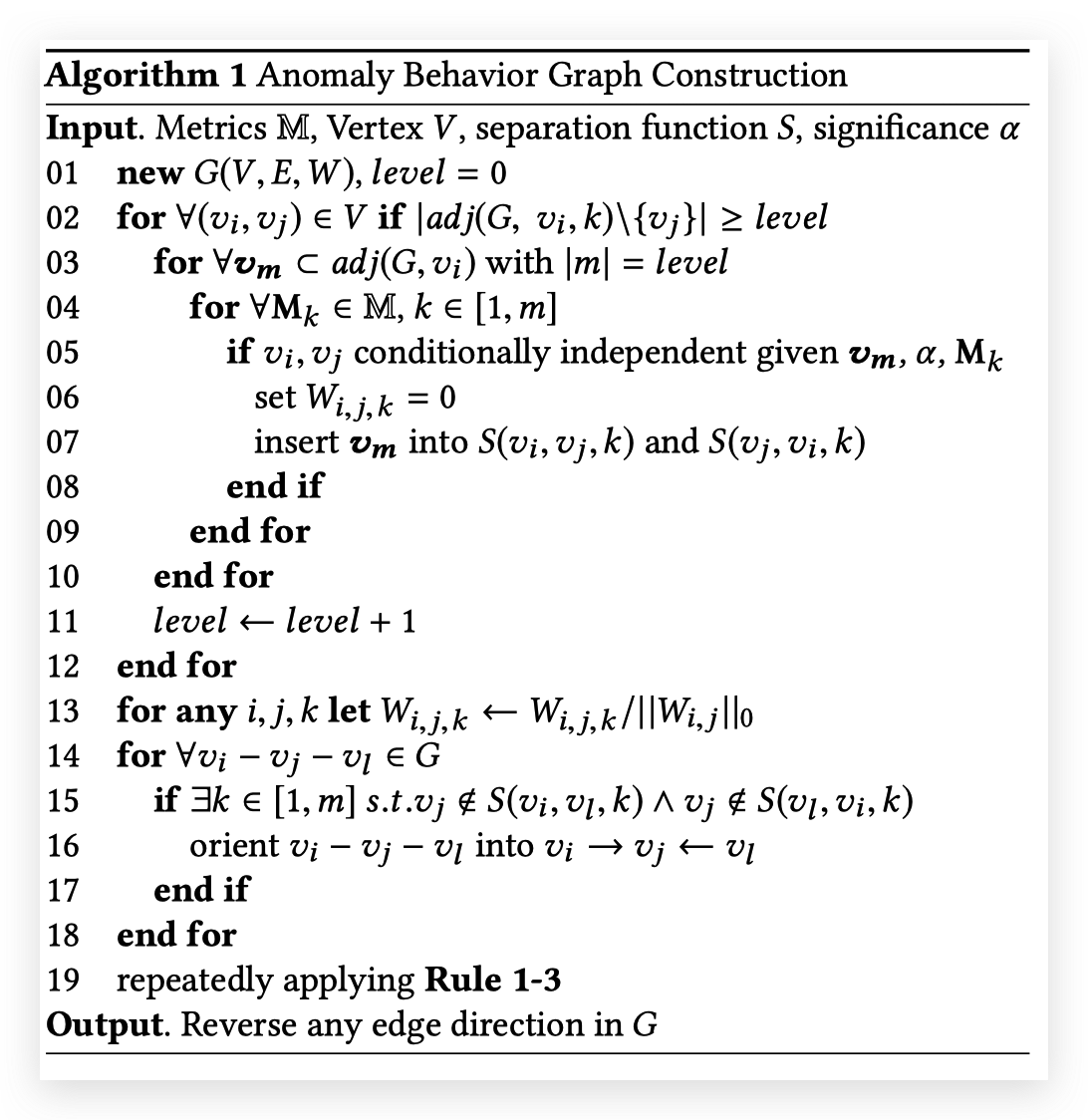#### 随机游走方法的改进：二阶游走¶

CloudRanger2对MonitorRank中采用的Personalized Page Rank提出了一种改进。
MonitorRank中使用的PPR，每一步的转移概率只和当前所在的节点有关:$$P_{i,j}=P[X_{t+1}=v_j|X_t=v_i]$$
CloudRanger则采用了二阶游走，认为转移概率和上一步转移的来源也有关:$$P_{i,j,k}=P[X_{t+1}=k|X_t=j, X_{t-1}=i]$$

#### 基于多指标的随机游走¶

MS-Rank3和AutoMAP9使用了多个指标进行随机游走，具体的方法把多指标得到的转移概率进行加权平均：$$P_{ij}\propto \sum_{m}W_{m, i, j}S_{m, j}$$，其中$$S_{m,j}$$是节点$$v_j$$和异常前端节点$$v_{fe}$$的指标$$m$$的相似度，$$W_{m,i,j}$$是节点$$v_i, v_j$$之间指标$$m$$的权重。

MS-Rank和AutoMAP使用了不同的思路去计算权重$$W_{m,i,j}$$，但是共同点是需要引入历史故障的定位结果。MS-Rank维护一个最佳的权重，AutoMAP会为每次定位计算一个最佳的权重。

MS-Rank计算了在历史数据中，基于每个单独的指标$$m$$定位的结果的精确率(top-k precision) $$P(m)$$。对于每个节点$$v_i$$$$W_{m,i,j}=W_{m, i}\propto P(m)$$，其中只计算$$v_i$$为根因的历史故障。这样的方法基本思想是，如果过去只通过指标$$m$$定位到$$v_i$$为根因的次数越多，那么就越依赖指标$$m$$。但是首先，这里$$W_{m,i}$$是计算从$$v_i$$向外转移时的指标权重，但是利用的却是$$v_i$$作为根因时的历史数据。其次，可能有的节点$$v_i$$从来都没有成为过根因，尤其是考虑到有准确根因记录的事件数量并不会很大。

AuotMAP中，$$W_{m,i,j}=W_{m}$$，即指标的权重和节点无关，每个指标全图只有一个权重$$W_m$$$$W_m$$通过历史故障数据中，和当前的依赖关系图（anomaly behavior graph）最相似的k个故障数据中的，根因节点和异常前端节点的指标$$m$$的相似度的加权平均得到，权重是每个故障的定位精确率。即$$W_m=\sum_{j=1}^{k}P(G_j)S_{m, rc_j}$$，其中$$G_j$$是每个故障的依赖关系图，$$P(G)$$表示使用$$G$$作为依赖关系图时的精确率。

#### 不同方法的对比和总结¶

1. 当故障发生时我们对于每一个异常前端都需要进行一次定位，并不是所有系统都有一个唯一的入口服务。
2. 如果当系统中同时出现两个故障时，每个后台服务可能会受到叠加的影响
3. 故障节点和异常前端节点之间经过的其他服务较多的时候，异常前端节点的指标中受故障节点的影响的比例可能会很小，导致它们的指标可能并不特别相似。这和具体的相似性计算方法也很有关系，上述方法应用的都是某种形式的perason correlation。
4. 指标相似和根因可能没有必然关系。例如这是我们在实际生产系统中遇到过的一种情况:调用关系为$$A->B->C$$，其中调用$$A\to B$$$$B\to C$$的响应时间都大大增加了，B和C响应时间都和A的响应时间是相似的。但是根因系统是$$B$$而不是随机游走的重点$$C$$，因为大部分的请求只到$$B$$就结束了，并没有进一步调用$$C$$

CloudRanger（使用延迟latency作为指标）和MS-Rank的结果完全相同。考虑两个方法十分相似，不同点只在于CloudRanger使用的是二阶游走，MS-rank使用了多指标综合，这两个方法使用的改进效果应该都比较有限。AutoMAP的结果比两者有少量提升，说明AutoMAP的多指标结合的方法更加有效。CloudRanger和MonitorRank的效果对比的话，只是略有提升，说明使用依赖关系图和调用关系图造成的影响也有限。

### 基于因果分析的方法¶

#### 基于因果图和调用链追溯根因¶

MicroScope7就采用了这样的方法，它利用PC算法（参见 基于因果分析进行随机游走）分析得到节点之间的依赖关系。当故障发生时，MicroScope从每条异常调用链的终端节点触发，利用上述原则，通过深度优先遍历（DFS）得到这一条调用链的根因节点。为什么不直接基于调用关系做这件事？因为调用关系和依赖关系有相交但是并不相等。共同依赖外部资源就是调用关系之外的依赖关系。

MicroScope采用的DFS的方法使得其只能分析无环的依赖关系调用链，但是节点之前完全可以有互相依赖的情况。

MicroScope的核心思想是分析出（在正常时刻）节点之间的依赖关系，然后只要一个节点的依赖有异常，那么就会认为它的异常是由依赖的节点导致的。

### 基于消失的相关关系的方法¶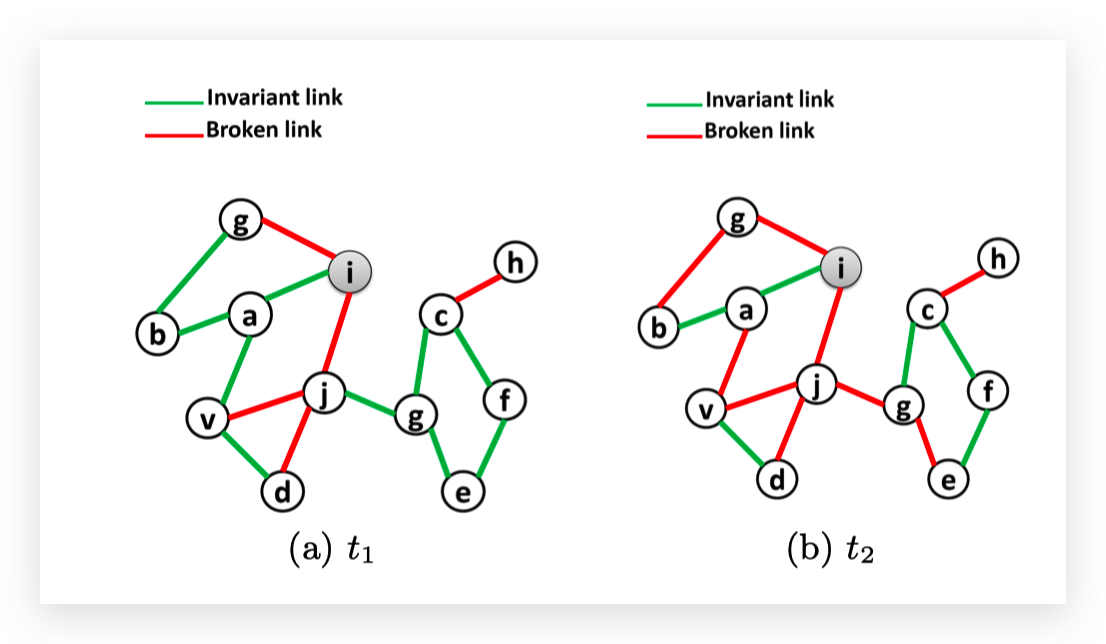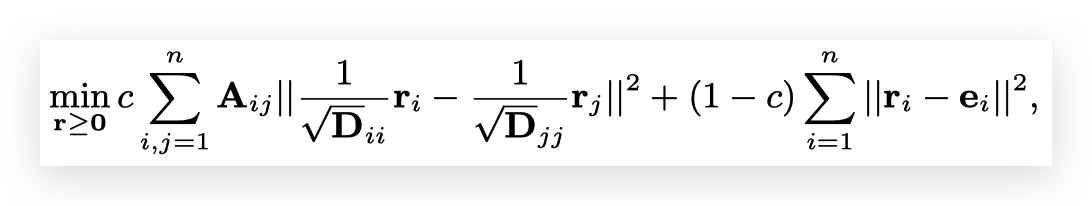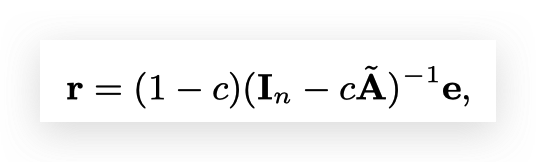RCA-KDD提出了一个保证收敛性的迭代算法解上述优化问题，并且在一个实际数据集上验证了性能。

CRD是对RCA-KDD的改进，主要的改进点是考虑节点会分成不同的组，故障会倾向于在组内传播。

RCA-KDD和CRD基于两个假设，提出了解决invariant network的有理论基础的有效方法。除了这两个假设在实际中的有效性之外，利用它们解决根因定位还有一个鸿沟，就是invariant network和实际的依赖关系并不相同。至少实际的依赖关系应该并不会是无向图（或者说是权重相同的双向的有向图）。

### 基于已知故障数据的有监督方法¶

#### 机器学习¶

MEPFL8把单条调用链的根因节点定位问题看作多分类问题（每个类别就是根因是某一个节点），使用端到端的有监督机器学习模型（随机森林，神经网络）解决这个问题。MEPFL的重点并不是机器学习，因此它只是简单地调用脸scikit-learning里面的KNN，RF和MLP这些包去做机器学习。MEPFL利用领域知识，将一个调用链编码为以下这些特征：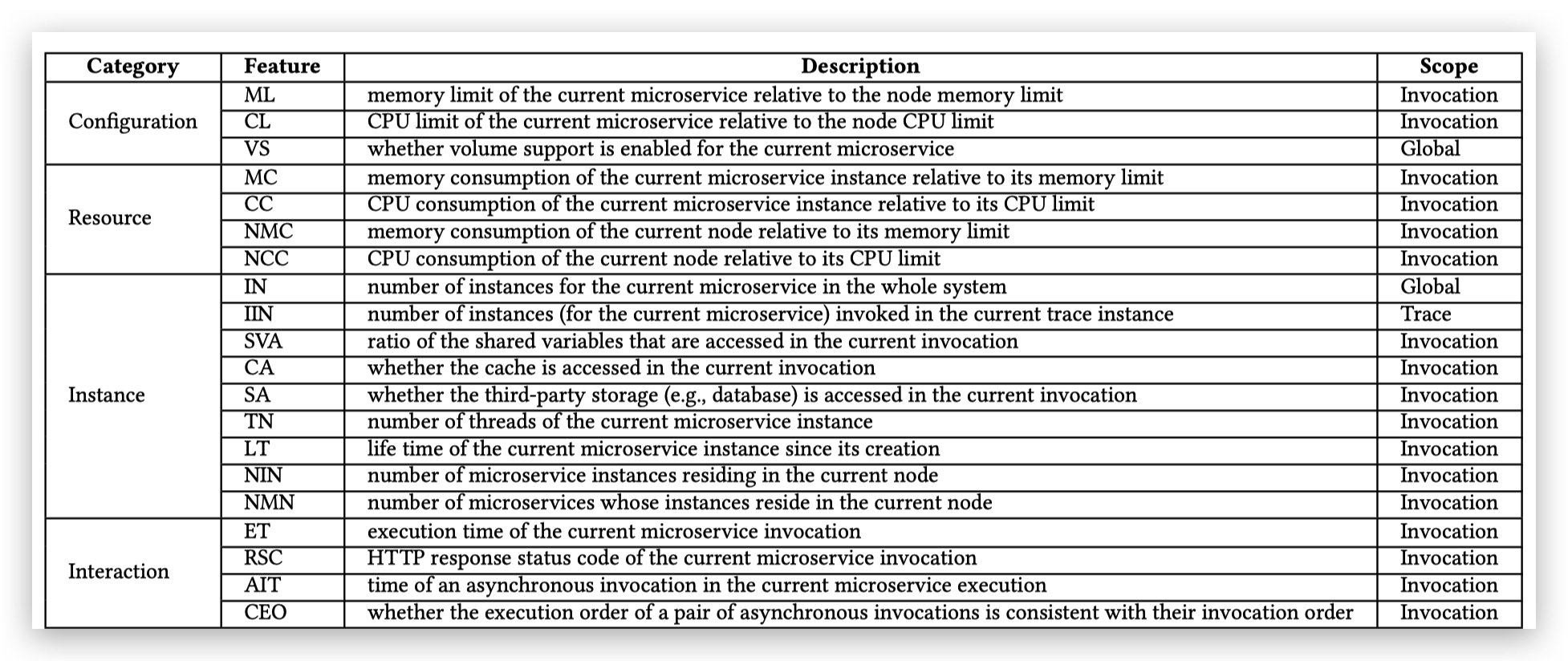MEPFL用的方法能取得非常好的效果。另外，从下图可以看出，MEPFL确实可以成功定位没有见过的异常调用链类型（一种特定故障注入在一个特定的微服务上是一种类型）。和MicroScope一样，MEPFL只处理单条调用链并不是实质性的问题。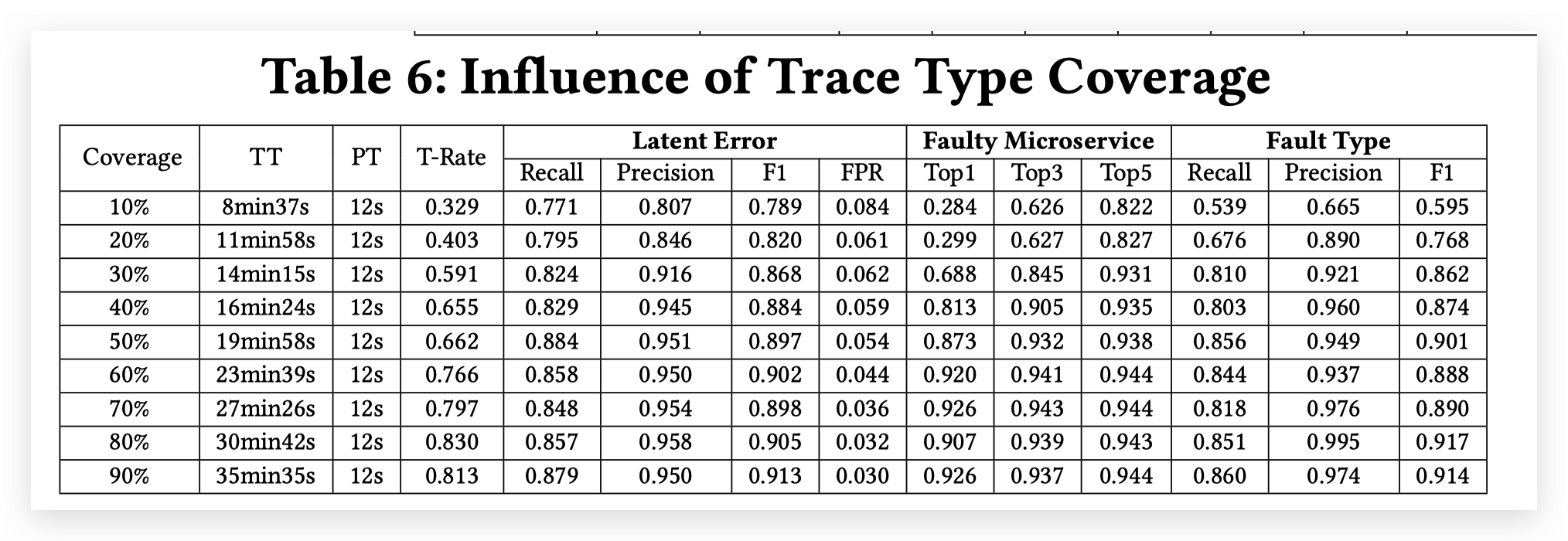#### 有监督方法的问题¶

• 半监督学习。通过引入部分领域知识，减少对标注数据的依赖。用了标注数据可以提升效果，没有的话效果也还过得去。AutoMAP这样的方法就有这种效果。
• 迁移学习。在一个完全受控制的测试系统上注入足够多类型故障，用这些数据训练模型。然后将其迁移到实际的生产系统上做检测。或者可以利用生产环境上少量的标注数据对模型进行微调以进一步提升效果。

### 基于关联规则挖掘的方法¶

RCSF1认为通过挖掘调用链路图上异常最集中的部分可以找到根因。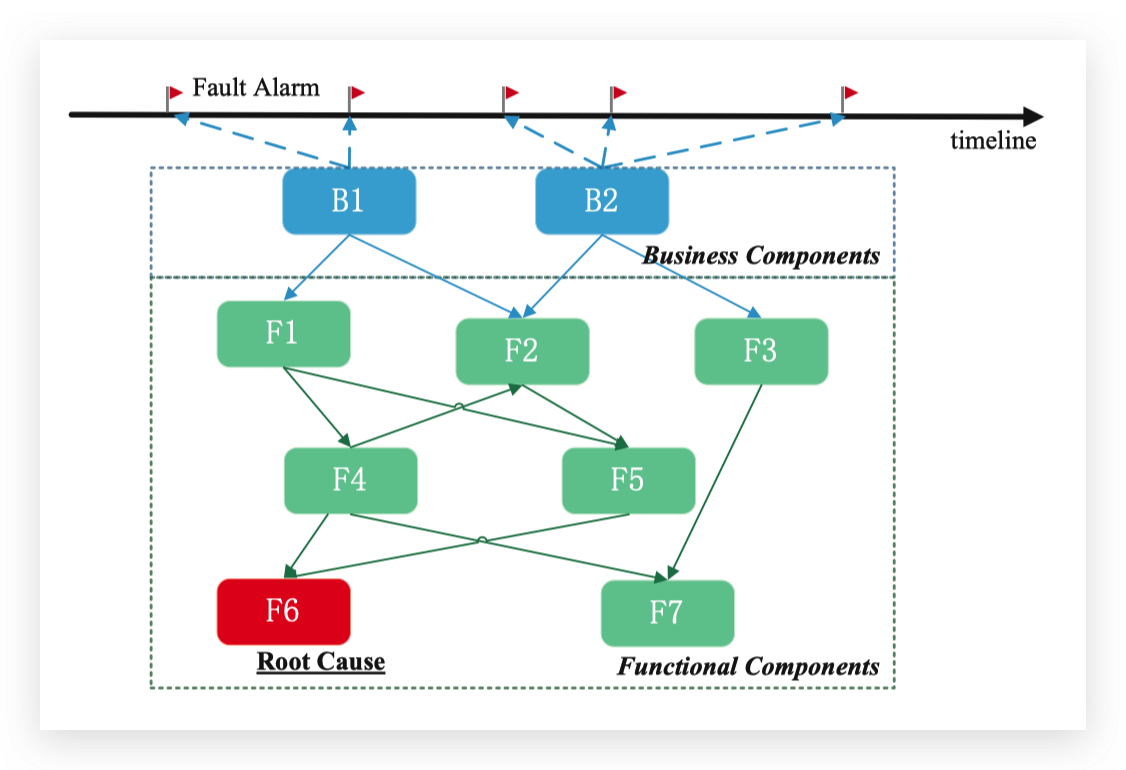RCSF的出发点很合理，但是RCSF生成的路径只是基于调用关系图的纸面路径而已，和实际的调用链路没有关系。

### 其他方法¶

RCA12中，将每一个节点的响应时间$$t_1, t_2, ..., t_n$$和调用链的总响应时间$$T$$建模为$$T=t_1+t_2+...+t_n$$。RCA将这个视为一个线性回归模型，通过LMG算法将线性回归的$$R^2$$分解到每一个节点的响应时间$$t_i$$上，即得到每一个节点的响应时间的变化对总响应时间的变化的贡献。RCA将其命名为相对重要性（relative importance）。

RCA通过每个节点的相对重要性对所有的节点排序，越重要的就越可能是根因。

## 参考文献¶

1. K. Wang et al., “A methodology for root-cause analysis in component based systems,” in 2015 IEEE 23rd International Symposium on Quality of Service (IWQoS), Jun. 2015, pp. 243–248, doi: 10.1109/IWQoS.2015.7404741

2. P. Wang et al., “CloudRanger: Root Cause Identification for Cloud Native Systems,” in 2018 18th IEEE/ACM International Symposium on Cluster, Cloud and Grid Computing (CCGRID), May 2018, pp. 492–502, doi: 10.1109/CCGRID.2018.00076

3. M. Ma, W. Lin, D. Pan, and P. Wang, “MS-Rank: Multi-Metric and Self-Adaptive Root Cause Diagnosis for Microservice Applications,” in 2019 IEEE International Conference on Web Services (ICWS), Jul. 2019, pp. 60–67, doi: 10.1109/ICWS.2019.00022

4. KimMyunghwan, SumbalyRoshan, and ShahSam, “Root cause detection in a service-oriented architecture,” ACM SIGMETRICS Performance Evaluation Review, Jun. 2013, doi: 10.1145/2494232.2465753

5. C. Pham et al., “Failure Diagnosis for Distributed Systems Using Targeted Fault Injection,” IEEE TRANSACTIONS ON PARALLEL AND DISTRIBUTED SYSTEMS, vol. 28, no. 2, p. 14, 2017.

6. J. Weng, J. H. Wang, J. Yang, and Y. Yang, “Root Cause Analysis of Anomalies of Multitier Services in Public Clouds,” IEEE/ACM Transactions on Networking, vol. 26, no. 4, pp. 1646–1659, Aug. 2018, doi: 10.1109/TNET.2018.2843805

7. J. Lin, P. Chen, and Z. Zheng, “Microscope: Pinpoint Performance Issues with Causal Graphs in Micro-service Environments,” in International Conference of Service-Oriented Computing, Cham, 2018, vol. 11236, pp. 3–20, doi: 10.1007/978-3-030-03596-9_1

8. X. Zhou et al., “Latent error prediction and fault localization for microservice applications by learning from system trace logs,” in Proceedings of the 2019 27th ACM Joint Meeting on European Software Engineering Conference and Symposium on the Foundations of Software Engineering, Tallinn, Estonia, Aug. 2019, pp. 683–694, doi: 10.1145/3338906.3338961

9. M. Ma, J. Xu, Y. Wang, P. Chen, Z. Zhang, and P. Wang, “AutoMAP: Diagnose Your Microservice-based Web Applications Automatically,” in Proceedings of The Web Conference 2020, Taipei, Taiwan, Apr. 2020, pp. 246–258, doi: 10.1145/3366423.3380111

10. G. Jeh and J. Widom. Scaling Personalized Web Search. In WWW, 2003.

11. Á. Brandón, M. Solé, A. Huélamo, D. Solans, M. S. Pérez, and V. Muntés-Mulero, “Graph-based root cause analysis for service-oriented and microservice architectures,” Journal of Systems and Software, vol. 159, p. 110432, Jan. 2020, doi: 10.1016/j.jss.2019.110432.

12. H. Jayathilaka, C. Krintz, and R. Wolski, “Performance Monitoring and Root Cause Analysis for Cloud-hosted Web Applications,” in Proceedings of the 26th International Conference on World Wide Web, Perth, Australia, Apr. 2017, pp. 469–478, doi: 10.1145/3038912.3052649

13. W. Cheng, K. Zhang, H. Chen, G. Jiang, Z. Chen, and W. Wang, “Ranking Causal Anomalies via Temporal and Dynamical Analysis on Vanishing Correlations,” in Proceedings of the 22nd ACM SIGKDD International Conference on Knowledge Discovery and Data Mining - KDD ’16, San Francisco, California, USA, 2016, pp. 805–814, doi: 10.1145/2939672.2939765

14. J. Ni et al., “Ranking Causal Anomalies by Modeling Local Propagations on Networked Systems,” in 2017 IEEE International Conference on Data Mining (ICDM), Nov. 2017, pp. 1003–1008, doi: 10.1109/ICDM.2017.129

Last update : July 1, 2023
Created : Jul 15, 2021